Certificate

### Learn on Scaler Topics and get certified.# Reverse a String in Java

Learn about Reverse a String in Java

## Overview

Strings are a sequence of characters in java. They are one of the most widely used data structures in java. Sometimes we will be required to reverse a string in java while programming. In this article, we will learn about various methods of reversing strings.

## Scope

• We will learn about reversing a string in java and why learning to reverse a string is important.
• We will learn about eight different methods to reverse a string using examples.

## Introduction to Reverse String in Java

Crosswords are fun and we all like the ones that make us think! When we solve a crossword, we fill it letter by letter to form a word, and eventually, the words help us to complete the entire grid.

Well if you have ever solved a crossword, it’s safe to say you have worked with characters and strings. Yes, you heard that right!A sequence of characters that act as a Java object is known as a string. After arrays, it is one of the most popularly used data structures. Basically, a string is a character array. If we think about a crossword again, the answer to the clue given, is nothing but a string and the letters forming the answer are characters.

Most operations can be done very easily on a character array. This would lead us to solve several string-based problems.(You can even code your own crossword using the multiple string operations available!)

In this tutorial, we will see the different techniques to reverse a string in Java. A string reversal might come in handy if you want to check whether a string is a palindrome or not. A palindrome string is a string that is equal when read from left to right and right to left.

It might even help if you are ever designing a crossword or a fun puzzle yourself!

### Consider the following example –

If the input string is “HELLO”, the reverse of it will be “OLLEH”. The given string is reversed but not a palindrome.

A good example of string palindromes is “aibohphobia”, which ironically enough is a fear of palindromes 😛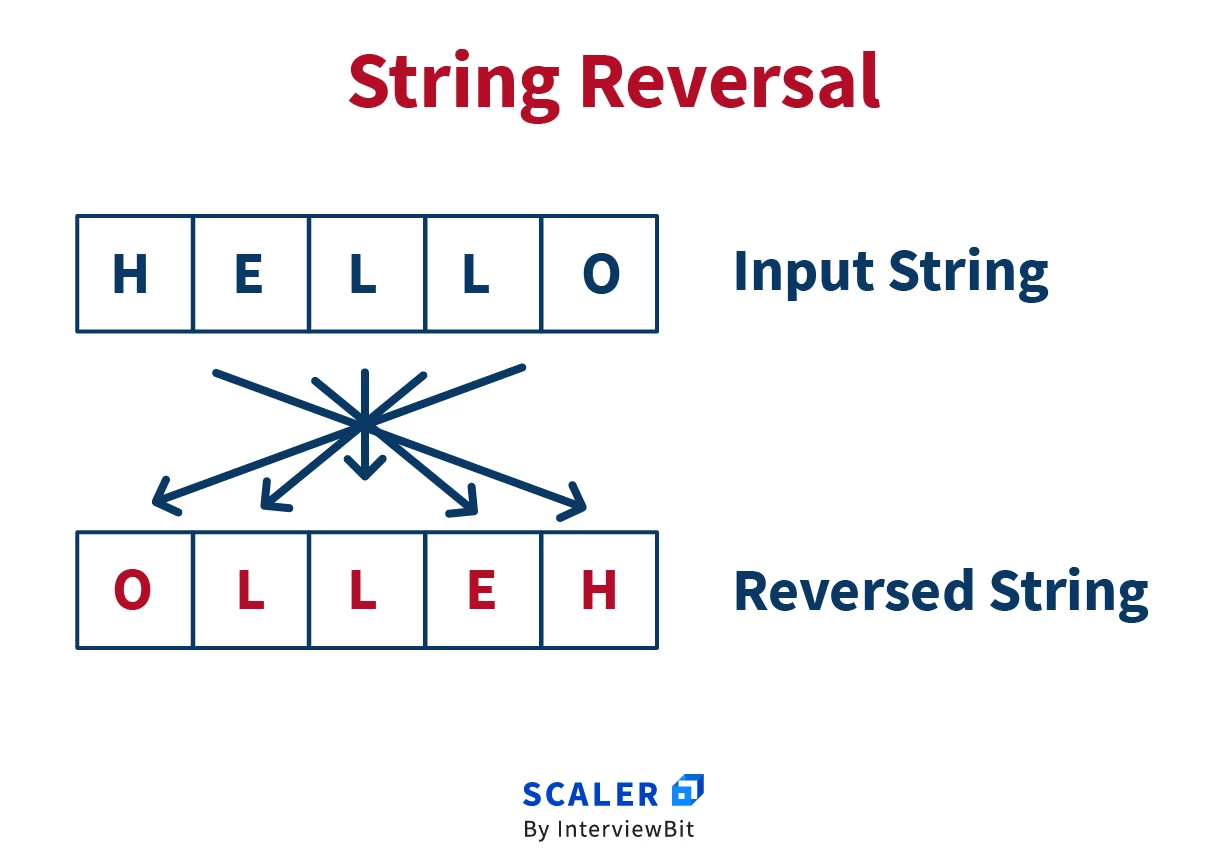## Different ways to reverse a string in Java

We are going to look into 8 different ways we can reverse a string in Java. Since strings are immutable i.e. they cannot be fundamentally changed, we always create a copy of the given string and then work on reversing them.

Every technique is coded and explained in the form of a function so that it can be easily implemented depending on the use case you are working with.

### 1. By using toCharArray()

Using the toCharArray() function is one way to reverse a string in Java.

``````public static String reverse(String str) {
String rev = "";
char[] finalarray = str.toCharArray();
for (int i = finalarray.length - 1; i >= 0; i--)
rev += finalarray[i];
return rev;
}
//Sample Output-"ymedacA relacS"
``````

### Let’s try to understand the above code –

• We have created a user-defined function called reverse().
• The toCharArray() is the function used to convert the string into a sequence of characters.
• After that, using the length() function, we are finding the length of the character array.
• We are printing the reverse of the character array using a for a loop.
• The time complexity of the code is O(N).
• The space complexity of the code is O(N).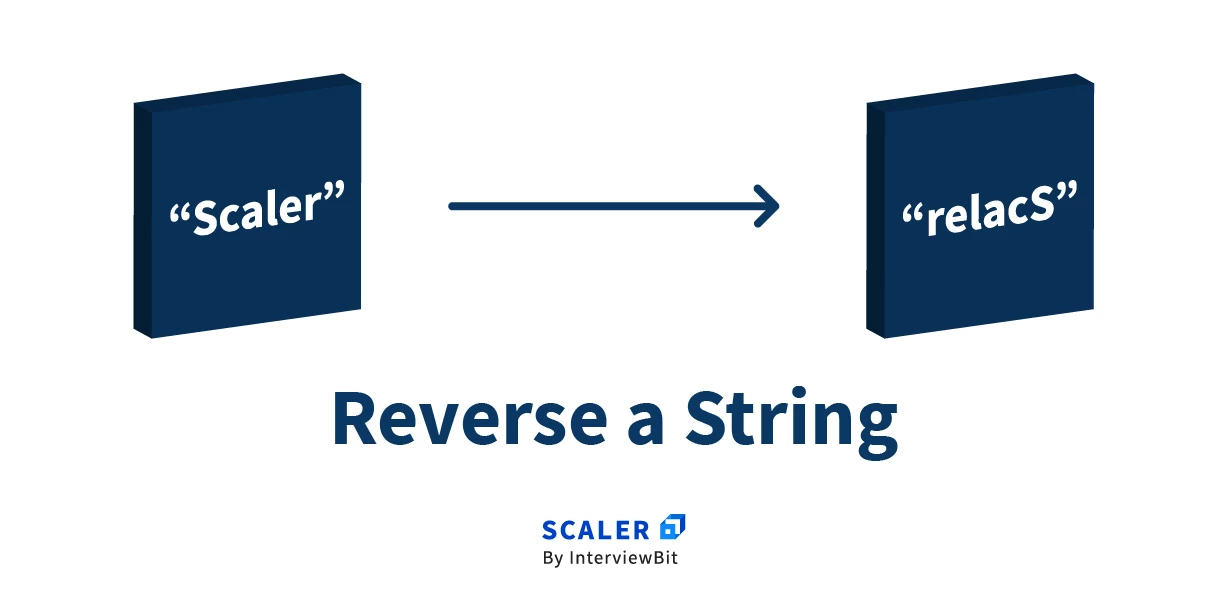### 2. By using StringBuilder

StringBuilder is a class in Java. It has an in-built method reverse(). This function reverses the characters in the string.

Take a look at the following code –

``````public String reverse(String str) {
StringBuilder obj = new StringBuilder();
//append is inbuilt method to append the data
obj.append(str);
obj.reverse();
return obj.toString();
}
//Sample Output-"ymedacA relacS"
``````
• StringBuilder objects are mutable. So we have created an object “obj” of the StringBuilder class.
• Next, we are appending the input string “str” using the append() method.
• The reverse() method is then called using obj and the result is returned.
• The time complexity of the code will be O(N).
• The space complexity of the code is O(N).

### 3. By using While Loop

One of the simplest ways to reverse a string in Java is to use the while loop. This approach works by adding the characters from the end to the beginning, to a new string variable- one character at a time.

``````public static String reverse(String str) {
String rev = "";
int len = str.length();
while (len > 0) {
rev += str.charAt(len - 1);
len--;
}
return rev;
}
//Sample Output-"ymedacA relacS"
``````

### Let’s try to understand the above code –

• We have used the length() method to find the length of the input string “str”.
• We run the while loop on the condition- length should be greater than zero.
• Next, we print the string using the charAt() method, which returns the character at the specified index of the given string.
• Lastly, we decrement the length variable “len”.
• The time complexity of the function is O(N).
• The space complexity of the function is O(N).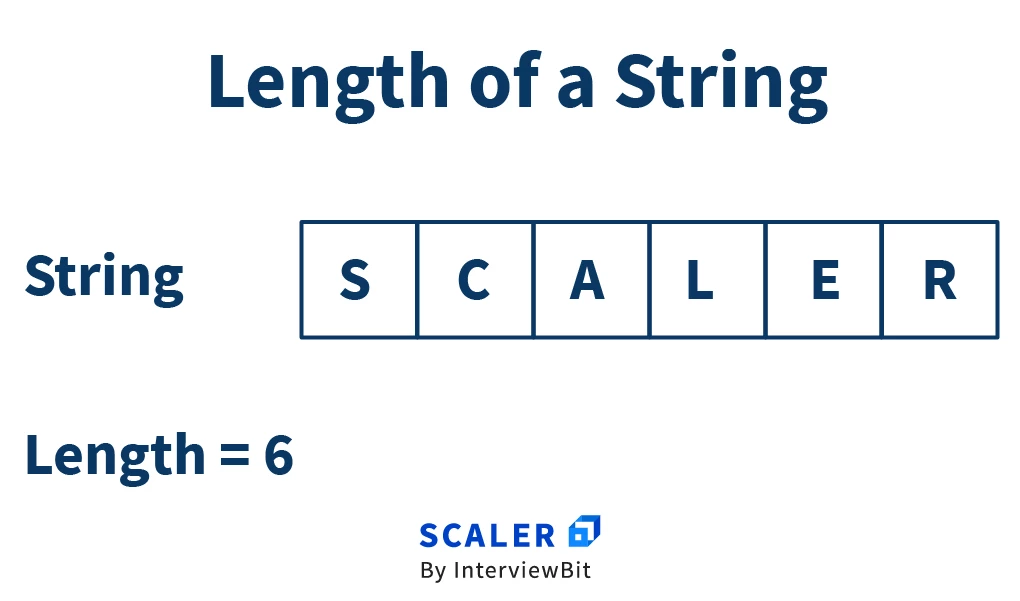### 4. By using For Loop

We can use a similar approach in the case of for loop, just like a while loop.

Let’s take a look –

``````public static String reverse(String str) {
String rev = "";
int i = 0;
int len = str.length();
for (i = len; i > 0; i--) {
rev += str.charAt(i - 1);
}
return rev;
}
//Sample Output-"ymedacA relacS"
``````
• We use the length method to find the length of the input string str.
• Next, we use a control variable i and then traverse the loop in reverse.
• We then use the charAt() function to create the string in a reverse order which is then returned.
• The time complexity of the function is O(N).
• The space complexity of the function is O(N).

### 5. By converting string to bytes

We can also use the getBytes() method to reverse a string in Java.

Let’s take a look how –

``````public static String reverse(String str) {
byte[] strAsByte = str.getBytes();
for (int l = 0, h = str.length() - 1; l < h; l++, h--) {
// swap values at `l` and `h`
byte temp = strAsByte[l];
strAsByte[l] = strAsByte[h];
strAsByte[h] = temp;
}
// convert byte array back into a string
return new String(strAsByte);
}
//Sample Output-"ymedacA relacS"
``````
• The getBytes() method is used to convert the input string str into Bytes.
• Next, we create a new temporary byte array whose length should be equal to the input string.
• We traverse the length of the byte array using a for a loop.
• We perform swapping inside the loop using a temp variable. The function eventually returns the reversed string strAsByte after converting it back to a string.
• The time complexity of the function is O(N).
• The space complexity of the function is O(N).

### 6. By using ArrayList objects

Let’s take a look at how you can reverse a string in Java using the ArrayList objects –

``````public static String reverse(String str) {
char[] strarray = str.toCharArray();
List < Character > revString = new ArrayList < > ();
for (char c: strarray)
Collections.reverse(revString);
return revString.toString();
}
//Sample Output-"ymedacA relacS"
``````

Now, let’s discuss the above code snippet and what are we achieving with it –

• Using, toCharArray() method, the string is converted into a character array.
• We add it to the ArrayList object.
• Next, we use the Collections class in Java which also has an in-built reverse() function.
• The ArrayList object revString is thus passed through this function to reverse it. The reversed string is then returned.
• The time complexity of the above function is O(N).
• The space complexity of the above function is O(N).

### 7. By using StringBuffer

Using the StringBuffer method, we can pretty efficiently reverse a string in Java.

Initially, we convert the input string to a StringBuffer and then use the in-built reverse() method to reverse the string.

The code snippet for it is as follows –

``````public static String reverse(String str) {
StringBuffer sb = new StringBuffer(str);
sb.reverse();
return sb.toString();
}
//Sample Output-"ymedacA relacS"
``````

In the above code, the time complexity of the function is O(N) and the space complexity is O(N).

### 8. By using recursion

Recursion is nothing but a function calling itself. So far we have been using looping structures to reverse a string in Java. But we can also achieve this by creating a recursive function.

One of the best yet quirky examples of recursion is when you type “recursion” as a Google Search.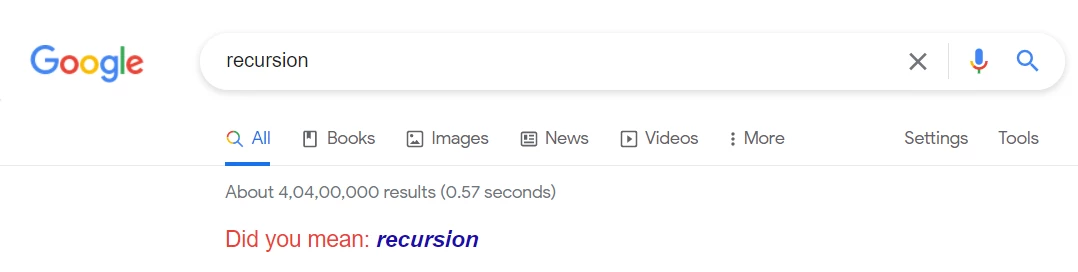As you can see, it asks you “Did you mean: recursion”, which is nothing but the repeated application of a procedure.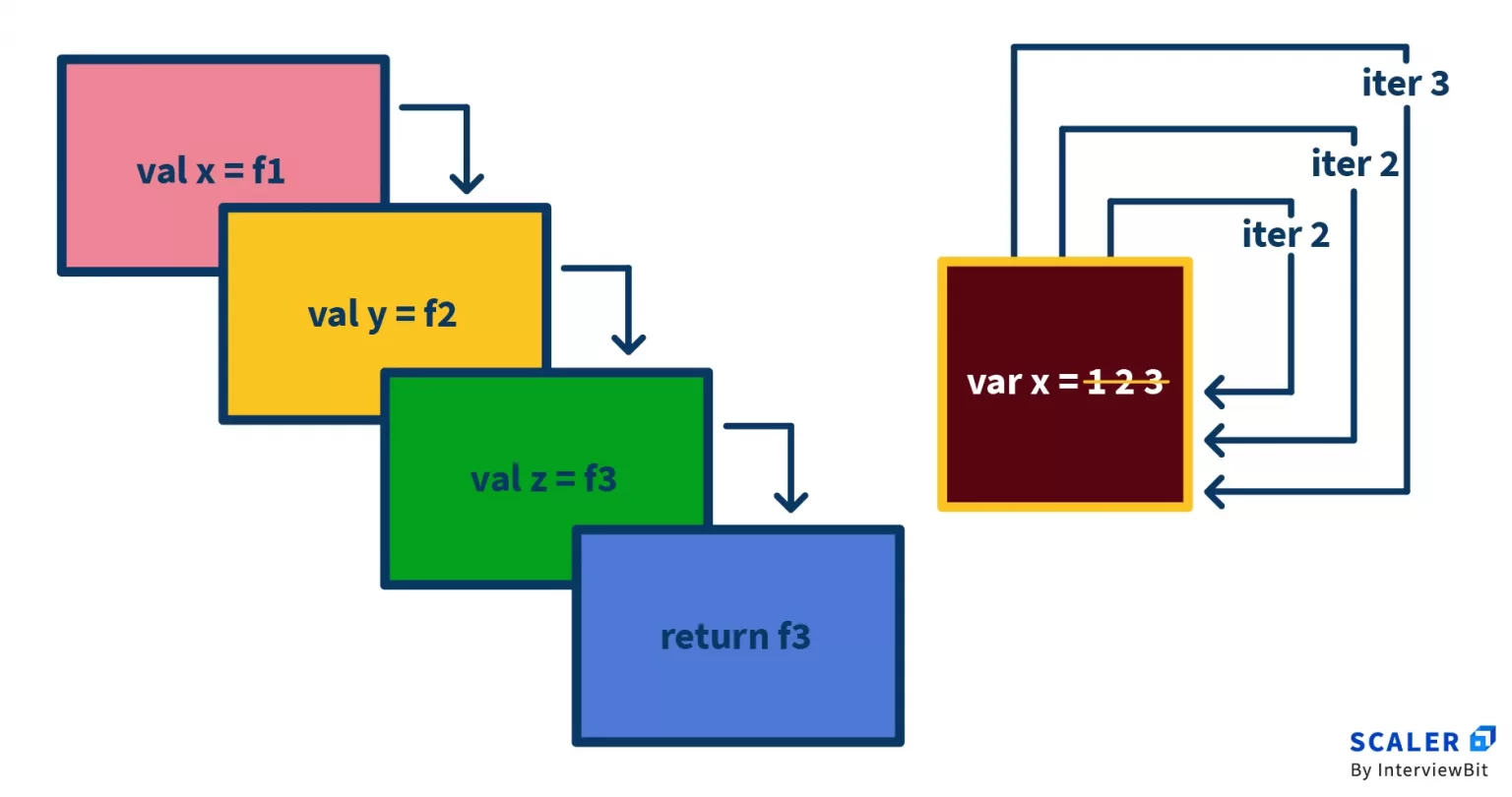So to reverse a string in Java, we will create a function that will be called recursively.

``````public static String reverse(String str) {
if (str.length() == 0)
return "";
return str.charAt(str.length() - 1) + reverse(str.substring(0, str.length() - 1));
}
``````
• In the above code snippet, we have created “reverse” as a recursive function.
• We pass the input string through the function.
• Using the substring() method, as you can see we have reversed the string easily in one line of code. That is the beauty of recursion.
• The time complexity of the function will be O(N^2) as we call the same function recursively for all the characters of the string.
• The space complexity will be O(N^2). This is so because the function is stored in the call stack. So every time substring() method works, it will return a copy of a start and end index, which also takes extra space.
• While iteration is a repetitive block of code, recursion gives us the advantage of writing shorter code. However, it has a higher time complexity than iteration structures. This is so because in recursion the allocation of a new stack frame takes place. So along with processing the iterations, it also has to deal with the recursive call stack frame, which makes it slower.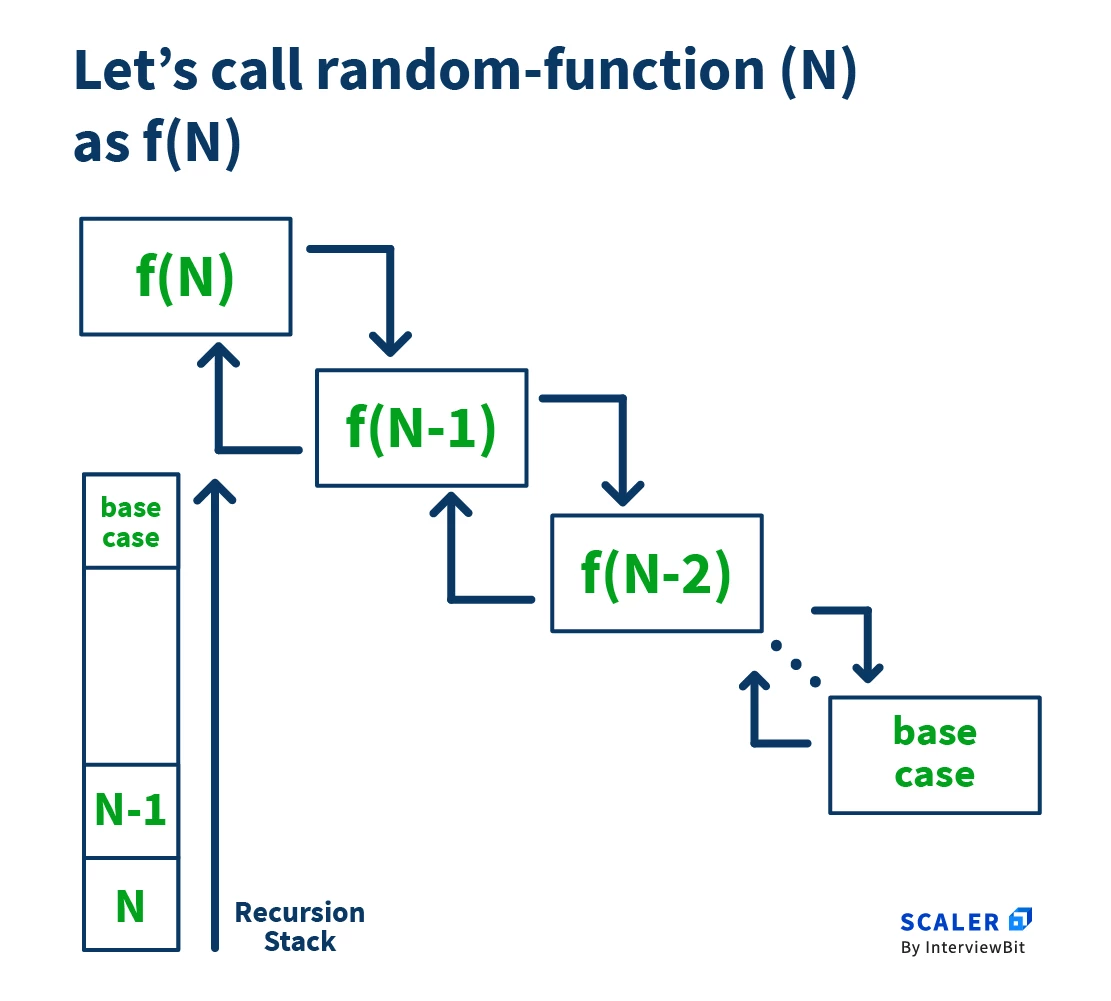## Conclusion

• Although Java string objects are fundamentally unchangeable, in this tutorial we saw the different ways we can manipulate them.
• In particular, we saw the different ways to reverse a string in Java.
• We can achieve this either by using in-built methods like reverse() or writing some logic to perform the string reversal. There are also several popular string functions in the String class that can be used to trim, compare, convert strings etc. After taking the time and space complexities into consideration, I would recommend the use of StringBuffer to reverse a string in Java. It offers the most favorable complexities and also has an easy-to-follow implementation.

Now, get coding and write your very own Java program to reverse a string!